Squares, roots and powers

This free course is available to start right now. Review the full course description and key learning outcomes and create an account and enrol if you want a free statement of participation.

Free course

2.6 Negative powers

Now look at what happens when the power is negative. What does 10−3 mean? What is the result of the following calculation?

100 ÷ 100 000

What you are actually being asked to find is: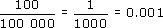But look at the calculation again. Using the rule for the division of powers of numbers gives:

102 ÷ 105 = 102−5 = 10−3

So 10−3 = 0.001. But you can also write this result as: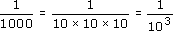This means that 10−3 can be thought of as 1 divided by 103.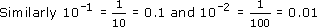This result is true for all negative powers, not just powers of ten. For example: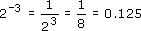One over any number is called the reciprocal of the number.

For example, the reciprocal of 10 is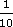= 10−1 and the reciprocal of 100 is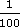=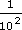= 10−2

A number raised to a negative power is the reciprocal of the number raised to the corresponding positive power.

So 10−5 =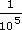= 0.00001.

Example 8

The decimal place value table columns are headed tenths, hundredths, thousandths etc. Write these as powers of ten.

a tenth ==10−1

a hundredth == 10−2

a thousandth =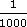= 10−3

So the headings of the place value table are all powers of ten. To the left of the units they are positive powers, the units column is 100, and to the right the column headings are negative powers of ten.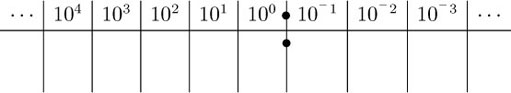Notice that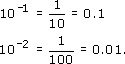The negative power indicates the position of the decimal point — how many times it has moved to the left from 100 = 1.

10−1 = 0.1 (move one to the left); 10−2 = 0.01 (move 2 to the left) etc.

MU120_4M4